## Representations of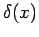.

In use, the Dirac delta function is never evaluated without multiplying by a test function and integrating over some domain. Equations involving Dirac delta functions without such integrations are a convenient half-way stage that nevertheless have enormous utility. Properly speaking, the Dirac delta function is not a function at all (it is a generalized function or a functional), however it can be represented as the limit of a sequence of ordinary functions.

Representations of the Dirac delta with familiar functions allow us to visualize the Dirac delta, and many calculations involvingcan be carried out with these representations. Let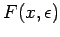be a function that has a peak near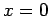, and the shape of the peak is controlled by parameter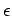. If the integral ofis unity, that is,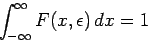for any value of parameter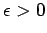, then the Dirac delta function may be represented in the limit: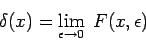Example representations:

1. Top-hat function (square step).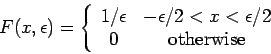2. Diffraction peak.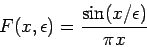3. Lorentzian.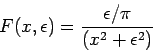4. Gaussian.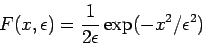5. Fourier integral. Using a standard integral, the Lorentz representation may be written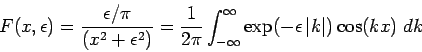and the limit can be explicitly evaluated to obtain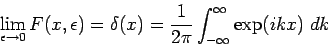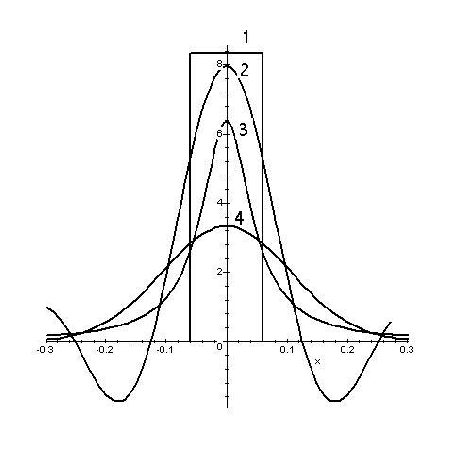NOTE: Although all of the above functionsare symmetric, symmetry is not essential. Non-symmetric functions produce perfectly good representations of the Dirac delta function.